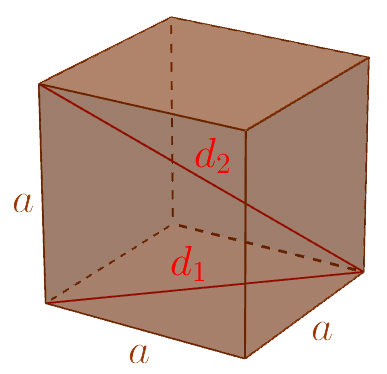# Cube

Keywords: cube, area, volume, side diagonal, volume diagonalArea

$A=6{a}^{2}$

Volume

$V={a}^{3}$

Side diagonal

${d}_{1}=\sqrt{2}a$

Volume diagonal

${d}_{2}=\sqrt{3}a$